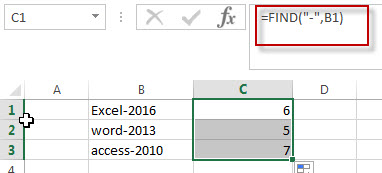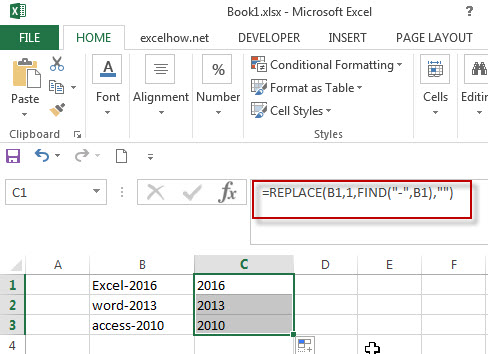# Combining the REPLACE Function with FIND Function to Remove Text in Excel

How to remove a text string or characters from a cell when the text is at a specific position. And this post will guide you how to remove a text string from a cell or column that the removed text is at a variable position in excel.

## Combining the Replace Function with Text Function to Remove Text

You can use FIND function to get the position of the specified character within the text string, and then combining within REPLACE function to remove the text. For example:

`=FIND("-"B1)`In the above formula, the FIND function will return the position of hyphen character (-) from the cell B1.

The Excel REPLACE function replaces all or part of a text string with another text string

The syntax of the REPLACE function is as below:

`= REPLACE(old_text, start_num, num_chars, new_text)`

If you want to remove the characters before the hyphen character, then you need use FIND function to get the position of hyphen in the text,  then combine with the REPLACE function and then we can write down the below formula:

`=REPLACE(B1,1,FIND("-",B1),"")`To remove text, you can use an empty string as “new_text” argument in REPLACE function.

### Related Functions

• Excel Replace function
The Excel DATE function returns the serial number for a date.The syntax of the DATE function is as below:= DATE (year, month, day) …
• Excel Find function
The Excel FIND function returns the position of the first text string (substring) from the first character of the second text string. The FIND function is a build-in function in Microsoft Excel and it is categorized as a Text Function….

Sidebar